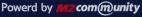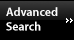Oh, Cha, Lee, Yu, Kim, Kim, and Kim: Validation of the Korean coefficient for the modification of diet in renal disease study equation

## Background/Aims:

Race and ethnicity are important determinants when estimatingglomerular filtration rate (GFR). The Korean coefficients for the isotope dilution mass spectrometry (IDMS) Modification of Diet in Renal Disease (MDRD) Study equations were developed in 2010. However, the coefficients have not been validated. The aim of this study was to validate the performance of the Korean coefficients for the IDMS MDRD Study equations.

## Methods:

Equation development and validation were performed in separate groups (development group, n = 147 from 2008 to 2009; validation group, n = 125 from 2010 to 2012). We compared the performance of the original IDMS MDRD equations and modified equations with Korean coefficients. Performance was assessed by comparing correlation coefficients, bias, and accuracy between estimated GFR and measured GFR, with systemic inulin clearance using a single injection method.

## Results:

The Korean coefficients for the IDMS MDRD equations developed previously showed good performance in the validation group. The new Korean coefficients for the four- and six-variable IDMS MDRD equations using both the development and validation cohorts were 1.02046 and 0.97300, respectively. No significant difference was detected for the new Korean coefficients, in terms of estimating GFR, between the original and modified IDMS MDRD Study equations.

## Conclusions:

The modified equations with Korean coefficients for the IDMS MDRD Study equations were not superior to the original equations for estimating GFR. Therefore, we recommend using the original IDMS MDRD Study equation without ethnic adjustment in the Korean population.

## INTRODUCTION

Chronic kidney disease (CKD) has become a global public health challenge due to various adverse outcomes, including accelerated cardiovascular events, all-cause mortality, and increased healthcare costs [1-3]. Early detection and timely intervention of CKD can prevent or delay these adverse outcomes . Glomerular filtration rate (GFR) is generally regarded as the best indicator of kidney function . The ideal method to assess GFR is by measuring clearance of an exogenous marker, such as inulin, but this is time-consuming, costly, and requires intensive labor. Therefore, equations to estimate GFR have been developed based on serum creatinine (SCr) and demographic factors such as age, sex, and body size [5,6]. Clinical guidelines recommend using the GFR estimating equation and reporting estimated GFR (eGFR) to recognize CKD early and properly manage the disease [7,8]. Reporting eGFR improves the detection rate of patients with CKD [9,10].
The isotope dilution mass spectrometry (IDMS) Modification of Diet in Renal Disease (MDRD) Study equation is widely used in clinical practice. However, race and ethnicity are important determinants when estimating GFR; thus, the MDRD Study equations derived for Caucasian and African Americans are less accurate in Asians [11,12]. To overcome this limitation, some Asian investigators have developed ethnic coefficients for these populations and explored applying the ethnic coefficients to the original MDRD Study equations to improve GFR estimation [11,13-15]. Although the ethnic coefficients were derived from the same Asian race, they differ considerably according to the ethnic population [11,14,15], which emphasizes the need for careful validation of each ethnicity-based coefficient.
Korean coefficients for the IDMS MDRD Study equations have also been reported . However, the coefficients have not been validated thoroughly. In this study, we validated the performance of the Korean coefficients for the IDMS MDRD Study equations derived from a development study by comparing them to the original equations to verity the previous results. In addition, we derived new Korean coefficients for more accurate GFR estimation using the combined development and validation dataset.

## Participants

The validation dataset included 125 subjects who participated in “The validation study for the Korean coefficient of eGFR,” which was a clinical study conducted at Seoul National University Hospital (SNUH) from 2010 to 2012. Healthy volunteers and patients with CKD who presented to the SNUH outpatient nephrology clinic were recruited for the study. All healthy volunteers had normal urinalysis results and had a measured GFR ≥ 60 mL/min/1.73 m2. The development dataset included 141 subjects who participated in the “Measurement of GFR and calculation of GFR estimates for Koreans” clinical study, which was conducted to develop an ethnic coefficient for the IDMS MDRD Study equation to estimate GFR more accurately in the Korean population . The validation dataset was used to validate the performance of the modified equations using Korean coefficients derived previously. A combined dataset of the validation and development data was used to derive new Korean coefficients for IDMS MDRD Study equations and to validate the performance of the modified equations with the new coefficients. The same inclusion and exclusion criteria were used for all participants in the development and validation datasets. Details of these criteria have been reported previously . Participants ≥ 18 years of age who agreed with the study objectives and voluntarily provided written informed consent were included.

## Glomerular filtration rate measurement

GFR was measured using systemic inulin clearance as the reference standard . Sinistrin (Inutest 25%; Fresenius Kabi Austria GmbH, Graz, Austria), an inulin analog, was used as a substitute for inulin because of its water solubility and ease of handling. The procedure was performed after an overnight fast, and participants were advised to avoid high integrity carbohydrate or caffeine-containing drinks, such as coffee, black tea, sugar, or juice. Two indwelling catheters, one for the inulin injection and the other for blood sampling, were inserted intravenously. An oral water load (10 mL/kg) was provided to produce appropriate urine flow 30 minutes before the inulin injection. A blank blood sample was drawn, and then the inulin was injected. We used the single short method to measure systemic inulin clearance . A total of 20 mL of Inutest (5 g sinistrin: equivalent to bonded fructose) was mixed with 30 mL normal saline, and the mixture was injected over 1 minute. Participants drank 250 mL water every 30 minutes. Eleven consecutive blood samples (5, 10, 15, 30, 60, 90, 120, 150, 180, 210, and 240 minutes after injection) were taken from the antecubital vein opposite to that used for the inulin injection. Each blood sample was centrifuged at 2,500 rpm for 10 minutes, and the serum was preserved at −80°C until analysis.
Inulin concentration (μg/mL) was measured by high-performance liquid chromatography (HPLC) . The HPLC system had a delivery system, an auto injector, and an ultraviolet detector (Gilson model 305/306 HPLC pumps, 234 auto injector, and 118 UV detector; Gilson, Villiers Le Bel, France). Measured GFR (mGFR) was determined by systemic inulin clearance, which was calculated as the infused dose divided by the total area under the plasma concentration-time curve obtained by curve fitting. A modified two-compartment pharmacokinetics model with zero-order administration of the dose over 1 minute was used to fit the plasma inulin decay curves [15,16]. Inulin clearance was normalized to a standard body surface area (BSA) of 1.73 m2 BSA, calculated with the Dubois-Dubois formula .

## Serum creatinine measurement

SCr values were measured using the alkaline picrate Jaffe kinetic method by a Hitachi 7600 analyzer (200FR; Toshiba, Tokyo, Japan) in a SNUH laboratory, and the assay was calibrated to standardized creatinine measured using the IDMS via corrected equations: calibrated SCr = 1.07 × measured SCr − 0.2 (mg/dL) until December 2010; and calibrated SCr = 1.00 × measured SCr − 0.3 (mg/dL) since January 2011.

## Ethics statement

This study protocol was approved by the Institutional Review Board (IRB) of the SNUH (IRB no. H-1006-012-320). Informed consent was confirmed by the IRB.

## Statistical analyses

All analyses and calculations were performed using the R version 2.15.1 (The Comprehensive R Archive Network: http://cran.r-project.org) and SAS version 9.1 (SAS Institute, Cary, NC, USA) software packages. We used GFR expressed on a log scale to obtain the ethnic coefficients for the IDMS MDRD Study equation and intended to simplify the models with the intercept forced to zero. As retransformation back to the usual scale could induce bias, the equations were adjusted using the smearing method . Data are presented as mean ± standard deviation. The difference between eGFR and mGFR was determined as eGFR – mGFR, and the absolute difference was defined as the absolute value of the calculated difference. The Wilcoxon signed-rank test was performed to compare the medians of the difference and absolute difference. Bias, precision, and overall accuracy were calculated to assess the relationship between mGFR and eGFR calculated using each equation. eGFR determined by each equation was compared with mGFR using the Bland-Altman analysis . Bias was expressed as the sum of the area between the axis-x and the Bland-Altman plot slopes. Precision was defined as the width between the 95% limits of agreement. Accuracy was determined as the percentage of eGFR that deviated < 15%, 30%, and 50% from the mGFR. The difference in accuracy between eGFRs using each equation was tested with the McNemar test. A p value < 0.05 was considered significant.

## Study population

Table 1 shows the clinical characteristics of the participants in each dataset. In total, 125 participants were included in the analysis to validate performance of the Korean coefficients for the IDMS MDRD Study equations derived previously . A total of 266 participants were included in the combined development and validation dataset to derive the new Korean coefficients and validate the equations. Mean ages in the validation and combined datasets were 50.2 ± 16.4 and 49.0 ± 15.8 years, respectively, and males comprised 63% and 58% of the groups, respectively. Both datasets included healthy volunteers (validation set, 24.0%; combined set, 19.5%) and patients with CKD. Mean SCr values were 1.51 ± 0.87 and 1.73 ± 1.01 mg/dL, and mean mGFR values were 61.0 ± 33.3 and 58.4 ± 31.6 mL/min/1.73 m2 in the validation and combined datasets, respectively.

## Validation of performance of the modified IDMS MDRD equations with Korean coefficients derived from a previous development study using the validation dataset

The GFR-estimating equations used in this study are listed in Table 2. The Korean coefficients derived from the previous study were 0.99096 and 0.95540 for the four-variable IDMS MDRD Study equation (4v IDMS MDRD) and the six-variable IDMS MDRD Study equation (6v IDMS MDRD), respectively . The GFR-estimating equation 0.99096 (Korean coefficient) × the 4v IDMS MDRD Study equation was designated equation 1, and 0.95540 (Korean coefficient) × 6v IDMS MDRD Study equation was designated equation 2. The overall performance of the modified IDMS MDRD Study equations with Korean coefficients (equations 1 and 2) was compared with those of the IDMS MDRD Study equations. Fig. 1 shows the correlations between mGFR and eGFR using the IDMS MDRD Study equations and the modified equations with Korean coefficients (equations 1 and 2). Linear regressions were made using mGFR against eGFR, and all of the eGFR values correlated well with mGFR (r2 = 0.80). When eGFR was plotted against mGFR, the eGFR slopes using the modified equations (equations 1 and 2) were not significantly different from those for eGFR using the IDMS MDRD Study equations.
eGFR on the Bland-Altman plot using the modified equations (equations 1 and 2) had less bias than eGFR using the IDMS MDRD Study equations, and the bias of the eGFR values using the 6v equations were less than those of eGFRs using the 4v equations (Table 3, Fig. 2). All of the equations underestimated GFR in subjects with GFR ≥ 60 mL/min/1.73 m2.
The median difference between eGFR and mGFR was smaller in the 4v modified equation (equation 1) compared with that in the IDMS MDRD Study equation, but the 6v equation showed the opposite result with a greater median difference in the modified equation (equation 2) than that in the IDMS MDRD Study equation. However, the medians of the absolute difference between eGFR and mGFR were not significantly different in any of the equations. In addition, the 15%, 30%, and 50% accuracy of eGFR using the modified equations (equations 1 and 2) were not significantly different compared with those of eGFR using the IDMS MDRD Study equation (Table 3). Furthermore, the performance results were generally consistent across subgroups defined by sex and age (data not shown).

## Development of new Korean coefficients and overall performance of the combined dataset

We derived new Korean coefficients for the IDMS MDRD Study equations for more precise estimates of GFR, including 266 participants from the development and validation combined dataset for the analysis. The final new coefficients to modify the 4v and 6v IDMS MDRD study equations for the Korean population, derived from all study datasets, were 1.02046 and 0.97300, respectively. Equation 3 was 1.02046 (Korean coefficient) × 4v IDMS MDRD Study equation, and equation 4 was 0.97300 (Korean coefficient) × 6v IDMS MDRD Study equation (Table 2).
The overall performance of the IDMS MDRD Study equations and the modified equations with the new Korean coefficients were examined using the combined dataset (Table 4). The correlations between mGFR and eGFR using the modified equations (equations 3 and 4) were compared to those using the IDMS MDRD Study equations. The scatterplots of eGFR against mGFR showed overall good correlations in all equations, and no significant slope differences were detected between eGFR values using the modified equations (equations 3 and 4) and those using IDMS MDRD Study equations (Table 4, Fig. 3).
The Bland-Altman plots showed good agreement between the mGFR and eGFR values using all equations (Fig. 4). The agreement appeared to be better for the 6v equations than for the 4v equations. The modified 4v equation (equation 3) had less bias than the MDRD IDMS Study equations, whereas the modified 6v equation (equation 4) had greater bias than the MDRD IDMS Study equations. Similarly, the median difference was smaller in the 4v modified equation and the 6v IDMS MDRD Study equation. However, the median absolute differences did not differ between any of the equations. The 15%, 30%, and 50% eGFR accuracy values using the modified equations did not improve compared with those for eGFR using the IDMS MDRD Study equations, except that the 15% eGFR accuracy using the 6v IDMS MDRD Study equation improved against the modified equation (55.2 vs. 52.8, p = 0.023). Analyses stratified according to sex and age showed similar results for all subgroups (data not shown).

## DISCUSSION

We previously reported Korean coefficients for the 4v and 6v IDMS MDRD Study equations (0.99096 and 0.9554, respectively), and demonstrated that eGFR using the modified IDMS MDRD Study equations with the ethnic coefficients was sufficient with respect to correlation with mGFR, bias, and accuracy, but the performance of the modified equations was not significantly different from that of the original IDMS MDRD Study equations . The present study verified our previous results and derived new Korean coefficients for the IDMS MDRD Study equations using a larger number of participants. eGFR using the modified IDMS MDRD Study equations with the previous ethnic coefficients showed a good correlation with mGFR determined by systemic inulin clearance in the validation dataset. The new Korean coefficients for the 4v and 6v IDMS MDRD Study equations derived from the combined development and validation dataset were 1.02046 and 0.97300, respectively. The modified equations showed good performance with the new ethnic coefficients, but no significant difference in the accuracy of estimating GFR was observed between the modified and original equations.
In both the previous and present studies, the ethnic coefficients for the IDMS MDRD Study equations were close to 1. The modified equations with the previous (0.99096) and new (1.02046) ethnic coefficients estimated 1% lower and 2% greater GFR, respectively, compared with that of the original equations in the Korean population. In other words, there was little difference in the GFR estimates regardless of whether the original IDMS MDRD Study equation or the modified equations with the ethnic coefficients were used, suggesting that using the original IDMS MDRD Study equations without adjusting for ethnicity to estimate GFR is reasonable in the Korean population.
Although the MDRD Study equation has been used globally in clinical practice and in many past studies, applying the equation to Asians, who were not included in the MDRD Study, has been questioned. Thus, Asian investigators have attempted to identify a method to more accurately estimate GFR by modifying the original MDRD Study equations, and ethnic coefficients from several Asian countries have been reported [11,13-15,21].
An absolute comparison of each ethnic coefficient is not reasonable because of methodological differences among Korean, Chinese, and Japanese studies, which may have been a potential source of bias and contributed to different results among them. Possible explanations for the discrepancies in ethnic coefficients among the three Asian ethnic groups are as follows. First, each study used a different method to measure reference GFR. Furthermore, the SCr value used to derive the Chinese coefficient was not calibrated to standardized Cr, unlike in the other studies. Subsequently, another investigator calculated a smaller Chinese coefficient (1.144)  than that of a previous study  using standardized SCr, but it was still greater than the Korean and Japanese coefficients. We measured GFR using systemic inulin clearance, but Chinese and Japanese studies used plasma clearance of technetium-99m diethylenetriaminepentaacetic acid (99mTc-DTPA) and urinary inulin clearance using the constant infusion method, respectively. Moreover, these methods are different from the renal clearance of 125I-iothalamate used in the MDRD Study. Several investigators have reported that iothalamate and 99mTc-DTPA clearance overestimate GFR compared with inulin clearance [22,23]. Using the bolus injection and constant infusion methods to determine inulin clearance may lead to differences in mGFR, even though several studies showed that the difference was small and would be acceptable in clinical practice [24,25]. Therefore, discrepancies between ethnic coefficients may be due to the different GFR measurement methods used in each study. Second, strategies for recruiting, and the clinical characteristics of the participants, were slightly different in each study. Our study was an outpatient-based study, whereas the Chinese study excluded participants with muscle atrophy, and the Japanese study was primarily inpatient-based. In addition, the causes of CKD were somewhat different among the studies. More than 50% of the participants in the Japanese study were patients with glomerulonephritis, and the proportion of participants with diabetes mellitus in the Chinese study was relatively smaller than in the other studies. The Chinese and Japanese coefficients were derived from patients diagnosed with CKD, but the present study included healthy volunteers without CKD. Previous studies have reported that patients with diabetes mellitus have super-normal GFR values at an early stage [26,27]. In addition, our results and those of the other studies showed that eGFR almost always underestimates mGFR in a healthy population [26,28]. Therefore, the different characteristics of the participants in these studies may have impacted on the derivation of the ethnic coefficients. Additionally, we cannot rule out the possibility that tubular secretion of Cr may differ among ethnic groups .
Our results indicate that it is reasonable to use the original IDMS MDRD Study equation for estimating GFR. This finding contrasts with the expectation that adjusting the original IDMS MDRD Study equation for ethnicity would improve the performance of GFR estimates, as demonstrated in previous studies by Asian investigators. However, Teo et al.  reported an ethnic coefficient of 1.027 for Indian/others in a multiethnic Asian population-based study, which is also close to 1 and differs little from the Korean coefficient. Teo et al.  showed that bias was different between Chinese and Indian/others, but not between Chinese and Malays. These results suggest that the extent of the ethnic impact on GFR estimates using the IDMS MDRD Study equation may differ according to the ethnic group, albeit ethnicity is an important factor to estimate GFR . In addition, this may be consistent with our finding that adjusting the original IDMS MDRD Study equation for ethnicity did not result in an appreciable difference in certain situations.
Several limitations of our study should be mentioned. First, the method we used to measure GFR was different from that used in the MDRD Study. We used systemic inulin clearance, whereas the MDRD Study equations were developed using renal clearance of 125I-iothalamate. Thus, the comparison between the original and modified equations may have been biased. Second, the size of the study population was relatively small compared with that used in previous studies of different racial and ethnic populations [5,6,11-14]. Therefore, we cannot rule out the possibility that the relatively smaller sample size may have affected the negative results (i.e., no significant difference between equations). In addition, we only included a few healthy volunteers without CKD. Considering that the difference between eGFR and mGFR was large among participants with GFR ≥ 90 mL/min/1.73 m2 in this study, our findings may not be generalizable to healthy individuals with normal renal function. Additionally, our study was restricted to participants with native kidneys. Clinical presentations, such as donated or recipient kidneys, influence the performance of known GFR-estimating equations [30,31]. Therefore, findings in transplant recipients may not be the same. Finally, new coefficients from the combined dataset should be validated in larger, more diverse populations.
In conclusion, the new Korean coefficients (1.02046/0.97300) for the IDMS MDRD Study equations were close to 1, and no significant difference in performance was detected between the modified equation and the original IDMS MDRD Study equation. Therefore, the original IDMS MDRD Study equation can be used to estimate GFR in Koreans without adjusting for ethnicity, because it is not only more convenient in clinical practice but does not produce any considerable difference.

## KEY MESSAGE

1. The new Korean coefficients for the four variable isotope dilution mass spectrometry (IDMS) Modification of Diet in Renal Disease (MDRD) Study equation and the six-variable IDMS MDRD Study equation were 1.02046 and 0.97300, respectively.
2. No significant difference was detected between the original IDMS MDRD Study equation and the modified equation when estimating glomerular filtration with the new Korean coefficients.

## Acknowledgments

This study was supported by a grant from the Korea Healthcare Technology R&D Project, Ministry for Health, Welfare, and Family Affairs, Republic of Korea (A070001).
##### Figure 1.
Correlation between estimated glomerular filtration rate (eGFR) and measured glomerular filtration rate (mGFR) in the validation dataset. mGFR was determined by systemic inulin clearance. Solid gray line indicates identical line, and solid blue line represents the fit line between eGFR and mGFR. eGFR was obtained using the (A) 4 variable isotope dilution mass spectrometry Modification of Diet in Renal Disease (4v IDMS MDRD) study equation, (B) 6 variable (6v) IDMS MDRD study equation, (C) modified 4v IDMS MDRD equation with Korean coefficient derived from development dataset (equation 1), and (D) modified 6v IDMS MDRD equation with Korean coefficient derived from development dataset (equation 2).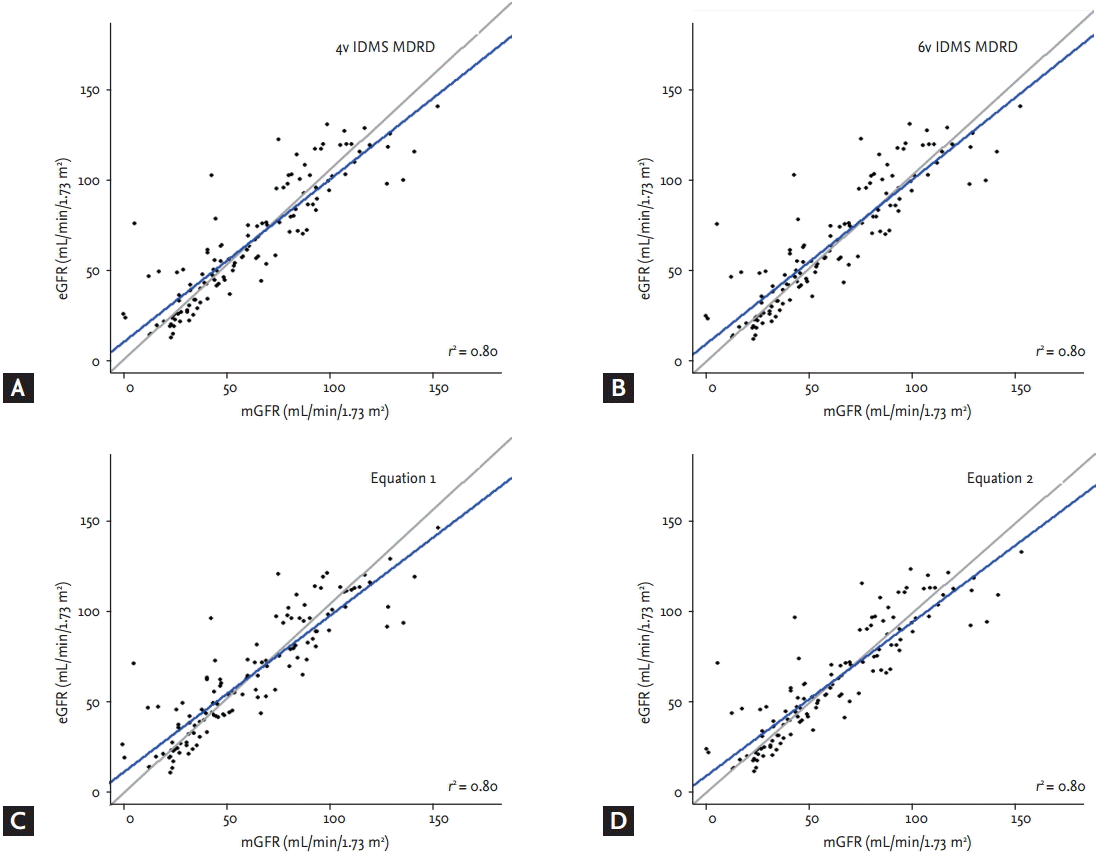##### Figure 2.
Bland-Altman plots between estimated glomerular filtration rate (eGFR) and measured glomerular filtration ration (mGFR) in the validation dataset. Solid gray line indicates the mean difference between eGFR and mGFR, dotted horizontal center line indicates no difference, and limits of agreement between two values (eGFR, mGFR) are indicated by the upper (mean + 2 SDs) and lower (mean - 2 SDs) dotted lines. Solid blue line represents the regression line between eGFR and mGFR. eGFR was obtained using the (A) 4 variable isotope dilution mass spectrometry Modification of Diet in Renal Disease (4v IDMS MDRD) study equation, (B) 6 variable (6v) IDMS MDRD study equation, (C) modified 4v IDMS MDRD equation with Korean coefficient derived form the development dataset (equation 1), and (D) modified 6v IDMS MDRD equation with Korean coefficient derived form the development dataset (equation 2).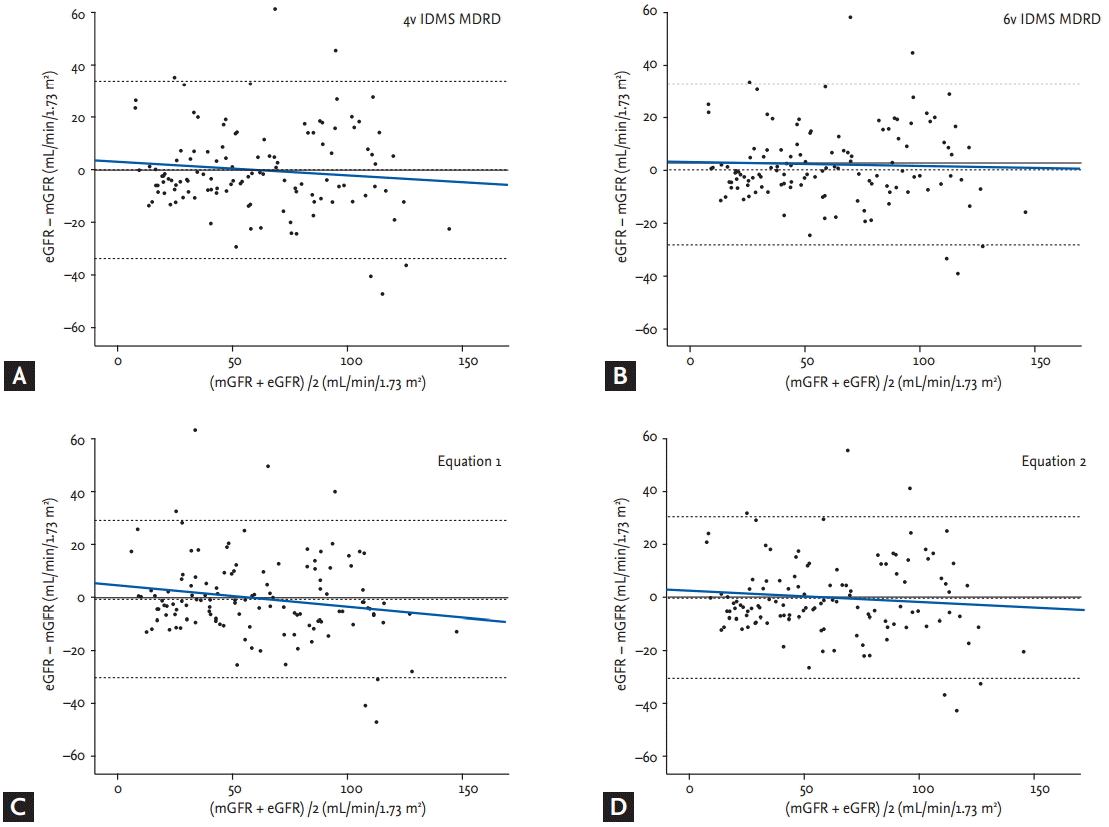##### Figure 3.
Correlation between estimated glomerular filtration rate (eGFR) and measured glomerular filtration rate (mGFR) in the combined dataset. mGFR was determined by systemic inulin clearance. Solid gray line indicates identical line, and solid blue line represents the fit line between eGFR and mGFR. eGFR was obtained using the (A) 4 variable isotope dilution mass spectrometry Modification of Diet in Renal Disease (4v IDMS MDRD) study equation, (B) 6 variable (6v) IDMS MDRD study equation, (C) modified 4v IDMS MDRD equation with Korean coefficient derived from the combined dataset (equation 3), and (D) modified 6v IDMS MDRD equation with Korean coefficient derived form the combined dataset (equation 4).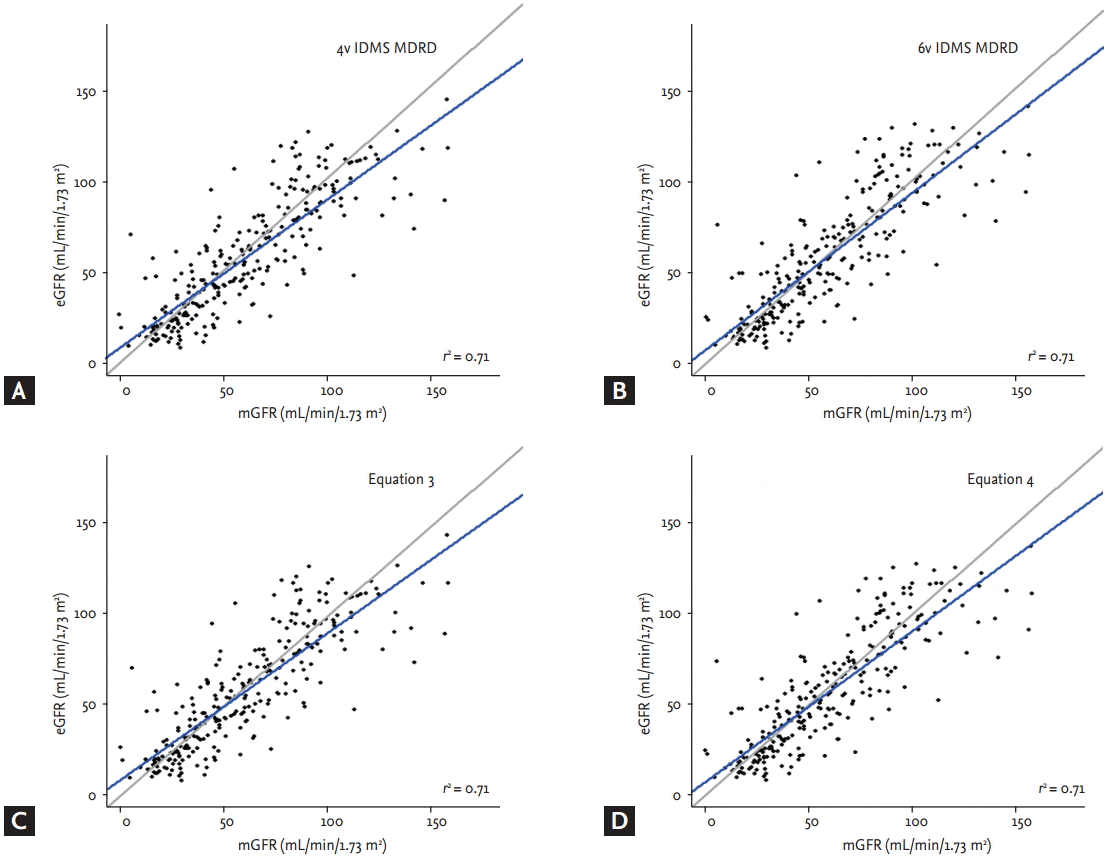##### Figure 4.
Bland-Altman plots between estimated glomerular filtration rate (eGFR) and measured glomerular filtration ration (mGFR) in the validation dataset. Solid gray line indicates the mean difference between eGFR and mGFR, dotted horizontal center line indicates no difference, and limits of agreement between two values (eGFR, mGFR) are indicated by the upper (mean + 2 SDs) and lower (mean - 2 SDs) dotted lines. Solid blue line represents the regression line between eGFR and mGFR. eGFR was obtained using the (A) 4 variable isotope dilution mass spectrometry Modification of Diet in Renal Disease (4v IDMS MDRD) study equation, (B) 6 variable (6v) IDMS MDRD study equation, (C) modified 4v IDMS MDRD equation with Korean coefficient derived form the combined dataset (equation 3), and (D) modified 6v IDMS MDRD equation with Korean coefficient derived form the combined dataset (equation 4).##### Table 1.
Clinical characteristics of the study population
Characteristic Combined dataset Validation dataset Development dataset
No. of subjects 266 125 141
Age, yr 49.0 ± 15.8 50.2 ± 16.4 47.9 ± 15.2
Male sex 154 (58) 79 (63) 75 (53)
Height, cm 164.0 ± 8.6 164.7 ± 8.5 163.3 ± 8.6
Weight, kg 65.4 ± 12.4 67.0 ± 12.9 64.0 ± 11.8
Body surface area, m2 1.72 ± 0.19 1.75 ± 0.19 1.69 ± 0.18
Body mass index, kg/m2 24.2 ± 3.6 24.6 ± 3.9 23.9 ± 3.4
Cause of chronic kidney disease
Diabetes mellitus 34 (12.8) 15 (12.0) 19 (13.5)
Hypertension 44 (16.5) 25 (20.0) 19 (13.5)
Glomerulonephritis 74 (27.8) 25 (20.0) 49 (34.8)
Others 62 (23.3) 30 (24.0) 32 (22.7)
Healthy volunteer 52 (19.5) 30 (24.0) 22 (15.6)
Hemoglobin, g/dL 13.0 ± 2.1 13.4 ± 2.0 12.7 ± 2.1
Albumin, g/dL 4.3 ± 0.4 4.3 ± 0.4 4.3 ± 0.4
Blood urea nitrogen, mg/dL 24.9 ± 16.3 21.9 ± 13.8 27.5 ± 17.9
Serum creatinine, mg/dL 1.73 ± 1.01 1.51 ± 0.87 1.92 ± 1.25
mGFR, mL/min/1.73 m2 58.4 ± 31.7 61.0 ± 33.3 55.9 ± 30.2
> 90 45 (16.9) 26 (20.8) 19 (13.5)
60-89 71 (26.7) 33 (26.4) 38 (27.0)
30-59 93 (35.0) 41 (32.8) 52 (36.9)
15-29 47 (17.7) 19 (15.2) 28 (19.9)
< 15 10 (3.8) 6 (4.8) 4 (2.8)

Values are presented as mean ± SD or number (%).

mGFR, measured glomerular filtration rate.

##### Table 2.
Intercepts and coefficients for the glomerular filtration rate-estimating equations used in the study
Equations Exponent transformed intercept Coefficient of continuous parameter
Exponent transformed coefficient of dichotomous variable
Serum creatinine Age Blood urea nitrogen Albumin Female Ethnicity
4V IDMS MDRD 175 -1.154 -0.203 - - 0.742 1.210 if Black
6v IDMS MDRD 161.5 -0.999 -0.176 -0.170 0.318 0.762 1.180 if Black
Equation 1 175 -1.154 -0.203 - - 0.742 0.99096 if Korean
Equation 2 161.5 -0.999 -0.176 -0.170 0.318 0.762 0.95540 if Korean
Equation 3 175 -1.154 -0.203 - - 0.742 1.02046 if Korean
Equation 4 161.5 -0.999 -0.176 -0.170 0.318 0.762 0.97300 if Korean

4v IDMS MDRD: four-variable IDMS MDRD study equation. 6v IDMS MDRD: six-variable IDMS MDRD study equation. Equation 1: 4v IDMS MDRD multiplied by the Korean coefficient derived from the development dataset. Equation 2: 6v IDMS MDRD multiplied by the Korean coefficient derived from the development dataset. Equation 3: 4v IDMS MDRD multiplied by the Korean coefficient derived from the combined dataset. Equation 4: 6v IDMS MDRD multiplied by the Korean coefficient derived from the combined dataset.

IDMS, isotope dilution mass spectrometry; MDRD, Modification of Diet in Renal Disease.

##### Table 3.
Overall performance of the glomerular filtration rate-estimating equations in the validation dataset
Equation Intercept (95% Cl) Slope (95% Cl) Adjusted R2 Difference, mL/min/1.73 m2 Absolute difference, mL/min/1.73 m2 Bias, AU Precision, mL/min/1.73m2 Accuracy, %
15% 30% 50%
4v IDMS MDRD 10.71 (5.75-15.67) 0.81 (0.74-0.88) 0.80 -2.2 (-8.1 to 6.9) 8.0 (3.2-14.3) 619.3 14.5 52.8 82.4 91.2
6v IDMS MDRD 9.68 (4.26-15.1) 0.88 (0.80-0.96) 0.80 -0.4 (-5.7 to 8.5) 6.9 (2.7-15.5) 315.1 15.3 55.2 84.8 92.0
Equation 1 10.93 (5.87—15.99) 0.83 (0.76-0.90) 0.80 -1.1 (-7.7 to 7.6) 7.7 (3.0-13.2) 457.4 14.7 52.0 83.2 90.4
Equation 2 9.42 (4.15-14.7) 0.86 (0.78-0.93) 0.80 -1.7 (-7.2 to 6.6) 7.1 (3.6-14.8) 258.0 15.1 52.8 84.8 92.0

Values are presented as median (25%–75%) unless other indicated. 4v IDMS MDRD: four-variable IDMS MDRD study equation. 6v IDMS MDRD: six-variable IDMS MDRD study equation. Equation 1: 4v IDMS MDRD multiplied by the Korean coefficient derived from the development dataset. Equation 2: 6v IDMS MDRD multiTable 2. Intercepts and coefficients for the glomerular filtration rate-estimating equations used in the study plied by the Korean coefficient derived from the development dataset.

CI, confidence interval; AU, arbitrary unit; IDMS, isotope dilution mass spectrometry; MDRD, Modification of Diet in Renal Disease.

##### Table 4.
Overall performance of the glomerular filtration rate-estimating equations in the combined dataset
Equation intercept (95% CI) Slope (95% CI) Adjusted R2 Difference, mL/min/1.73 m2 Absolute difference, mL/min/1.73 m2 Bias, AU Precision,mL/min/1.73m2 Accuracy, %
15% 30% 50%
4v IDMS MDRD 8.19 (4.07-12.31) 0.8 (0.74-0.86) 0.71 -3.6 (-11.4 to 4.9) 9.1 (4.1-17.9) 654.7 17.5 41.0 70.3 86.5
6v IDMS MDRD 7.39 (3.04-11.74) 0.86 (0.79-0.92) 0.71 -1.8 (-9.2 to 7.5) 8.1 (4.3-18.6) 119.7 17.9 42.9a 72.9 86.8
Equation 3 8.36 (4.15-12.56) 0.82 (0.75-0.88) 0.71 -2.6 (-10.3 to 5.8) 8.8 (3.9-17.9) 422.9 17.6 40.6 71.1 86.5
Equation 4 7.19 (2.96-11.42) 0.83 (0.77-0.90) 0.71 -3.1 (-10.5 to 5.8) 8.7 (4.3-18.6) 417.5 17.6 40.6 72.2 87.6

Values are presented as median (25%–75%) unless other indicated. 4v IDMS MDRD: four-variable IDMS MDRD study equation. 6v IDMS MDRD: six-variable IDMS MDRD study equation. Equation 3: 4v IDMS MDRD multiplied by the Korean coefficient derived from the combined dataset. Equation 4: 6v IDMS MDRD multiplied by the Korean coefficient derived from the combined dataset.

CI, confidence interval; AU, arbitrary unit; IDMS, isotope dilution mass spectrometry; MDRD, Modification of Diet in Renal Disease.

a p < 0.05, compared with equation 4

## REFERENCES

1. Eknoyan G, Lameire N, Barsoum R, et al. The burden of kidney disease: improving global outcomes. Kidney Int 2004;66:1310–1314.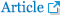2. Go AS, Chertow GM, Fan D, McCulloch CE, Hsu CY. Chronic kidney disease and the risks of death, cardiovascular events, and hospitalization. N Engl J Med 2004;351:1296–1305.3. Lina CY, WeiLinaa J. Association of Framingham Risk Score with chronic kidney disease: insight from National Health and Nutrition Examination Survey 2003-2006. Kidney Res Clin Pract 2012;31:A52.4. Huisman RM. The deadly risk of late referral. NephrolDial Transplant 2004;19:2175–2180.5. Levey AS, Bosch JP, Lewis JB, Greene T, Rogers N, Roth D. A more accurate method to estimate glomerular filtration rate from serum creatinine: a new prediction equation. Modification of Diet in Renal Disease Study Group. Ann Intern Med 1999;130:461–470.6. Levey AS, Coresh J, Greene T, et al. Using standardized serum creatinine values in the modification of diet in renal disease study equation for estimating glomerular filtration rate. Ann Intern Med 2006;145:247–254.7. National Kidney Foundation. K/DOQI clinical practice guidelines for chronic kidney disease: evaluation, classification, and stratification. Am J Kidney Dis 2002;39(2 Suppl 1):S1–S266.8. Levey AS, Eckardt KU, Tsukamoto Y, et al. Definition and classification of chronic kidney disease: a position statement from Kidney Disease: Improving Global Outcomes (KDIGO). Kidney Int 2005;67:2089–2100.9. Wang V, Maciejewski ML, Hammill BG, et al. Recognition of CKD after the introduction of automated reporting of estimated GFR in the Veterans Health Administration. Clin J Am Soc Nephrol 2014;9:29–36.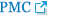10. Kagoma YK, Weir MA, Iansavichus AV, et al. Impact of estimated GFR reporting on patients, clinicians, and health-care systems: a systematic review. Am J Kidney Dis 2011;57:592–601.11. Ma YC, Zuo L, Chen JH, et al. Modified glomerular filtration rate estimating equation for Chinese patients with chronic kidney disease. J Am Soc Nephrol 2006;17:2937–2944.12. Imai E, Horio M, Nitta K, et al. Estimation of glomerular filtration rate by the MDRD study equation modified for Japanese patients with chronic kidney disease. Clin Exp Nephrol 2007;11:41–50.13. Matsuo S, Imai E, Horio M, et al. Revised equations for estimated GFR from serum creatinine in Japan. Am J Kidney Dis 2009;53:982–992.14. Imai E, Horio M, Nitta K, et al. Modification of the Modification of Diet in Renal Disease (MDRD) Study equation for Japan. Am J Kidney Dis 2007;50:927–937.15. Lee CS, Cha RH, Lim YH, et al. Ethnic coefficients for glomerular filtration rate estimation by the Modification of Diet in Renal Disease study equations in the Korean population. J Korean Med Sci 2010;25:1616–1625.16. Prescott LF, Freestone S, McAuslane JA. Reassessment of the single intravenous injection method with inulin for measurement of the glomerular filtration rate in man. Clin Sci (Lond) 1991;80:167–176.17. Pastore A, Bernardini S, Dello Strologo L, Rizzoni G, Cortese C, Federici G. Simultaneous determination of inulin and p-aminohippuric acid in plasma and urine by reversed-phase high-performance liquid chromatography. J Chromatogr B Biomed Sci Appl 2001;751:187–191.18. Du Bois D, Du Bois EF. A formula to estimate the approximate surface area if height and weight be known. 1916. Nutrition 1989;5:303–311.19. Duan N. Smearing estimate: a nonparametric retransformation method. J Am Stat Assoc 1983;78:605–610.20. Bland JM, Altman DG. Statistical methods for assessing agreement between two methods of clinical measurement. Lancet 1986;1:307–310.21. Teo BW, Xu H, Wang D, et al. GFR estimating equations in a multiethnic Asian population. Am J Kidney Dis 2011;58:56–63.22. Waller DG, Keast CM, Fleming JS, Ackery DM. Measurement of glomerular filtration rate with technetium-99m DTPA: comparison of plasma clearance techniques. J Nucl Med 1987;28:372–377.23. Perrone RD, Steinman TI, Beck GJ, et al. Utility of radioisotopic filtration markers in chronic renal insufficiency: simultaneous comparison of 125I-iothalamate, 169Yb-DTPA, 99mTc-DTPA, and inulin: the Modification of Diet in Renal Disease Study. Am J Kidney Dis 1990;16:224–235.24. van Rossum LK, Cransberg K, de Rijke YB, Zietse R, Lindemans J, Vulto AG. Determination of inulin clearance by single injection or infusion in children. Pediatr Nephrol 2005;20:777–781.25. van Rossum LK, Mathot RA, Cransberg K, Vulto AG. Optimal sampling strategies to assess inulin clearance in children by the inulin single-injection method. Clin Chem 2003;49:1170–1179.26. Vervoort G, Willems HL, Wetzels JF. Assessment of glomerular filtration rate in healthy subjects and normoalbuminuric diabetic patients: validity of a new (MDRD) prediction equation. Nephrol Dial Transplant 2002;17:1909–1913.27. Rossing P, Rossing K, Gaede P, Pedersen O, Parving HH. Monitoring kidney function in type 2 diabetic patients with incipient and overt diabetic nephropathy. Diabetes Care 2006;29:1024–1030.28. Poggio ED, Wang X, Greene T, Van Lente F, Hall PM. Performance of the modification of diet in renal disease and Cockcroft-Gault equations in the estimation of GFR in health and in chronic kidney disease. J Am Soc Nephrol 2005;16:459–466.29. Hsu CY, Chertow GM, Curhan GC. Methodological issues in studying the epidemiology of mild to moderate chronic renal insufficiency. Kidney Int 2002;61:1567–1576.30. White CA, Akbari A, Doucette S, Fergusson D, Knoll GA. Estimating glomerular filtration rate in kidney transplantation: is the new chronic kidney disease epidemiology collaboration equation any better? Clin Chem 2010;56:474–477.31. Murata K, Baumann NA, Saenger AK, Larson TS, Rule AD, Lieske JC. Relative performance of the MDRD and CKDEPI equations for estimating glomerular filtration rate among patients with varied clinical presentations. Clin J Am Soc Nephrol 2011;6:1963–1972.TOOLS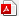PDF Links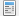PubReader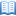ePub LinkFull text via DOI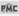Full text via PMCDownload CitationCrossRef TDM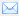E-Mail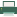PrintShare:METRICS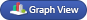2 Crossref
 2 Scopus
 9,052 View